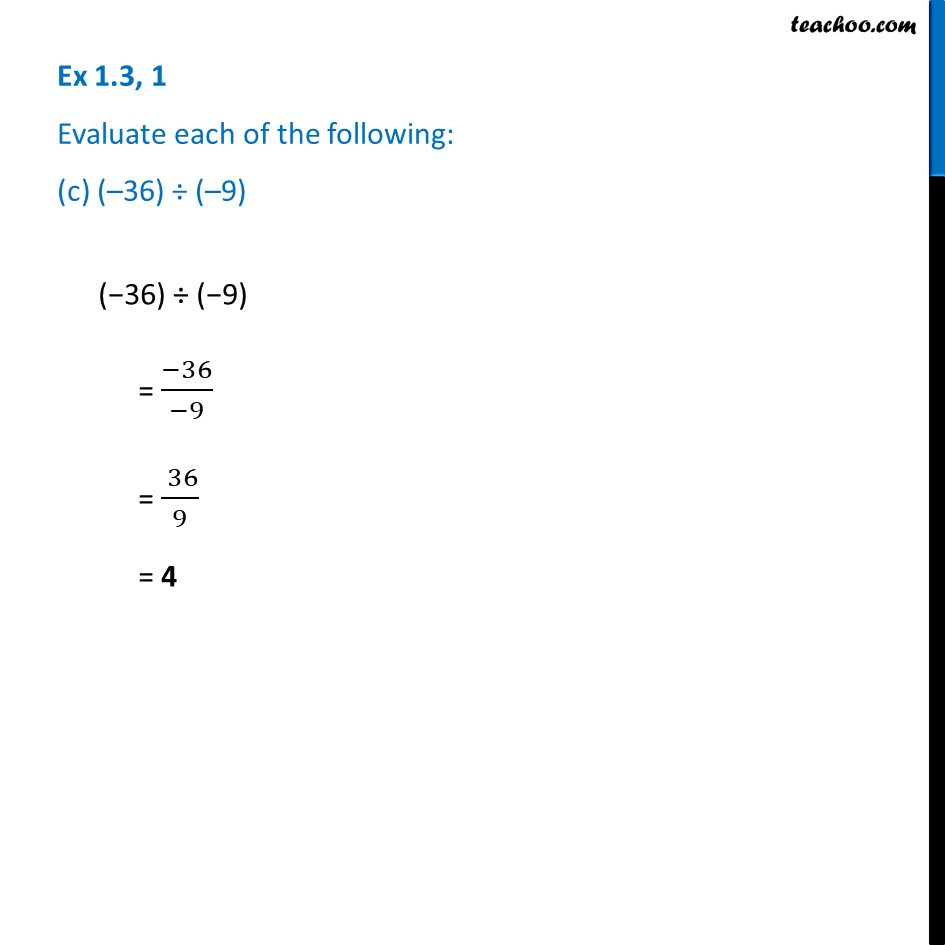Ex 1.3

Chapter 1 Class 7 Integers
Serial order wiseLearn in your speed, with individual attention - Teachoo Maths 1-on-1 Class

### Transcript

Ex 1.3, 1 Evaluate each of the following: (c) (–36) ÷ (–9)(−36) ÷ (−9) = (−36)/(−9) = ( 36)/9 = 4